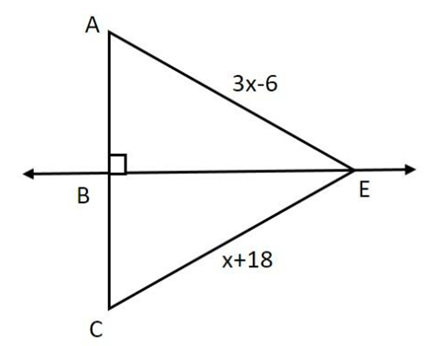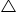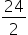Maths-
General
Easy

Question

# If 𝐴𝐵 ≅ 𝐵𝐶, Find 𝐶𝐸.Hint:

## The correct answer is: Hence, CE = 30 units.

### Answer: Step by step explanation:  Given: AE = 3x - 6.CE = x + 18.AB = BCABE = CBE = 90oTherefore, BE is perpendicular bisector at BC. Step 1: InACE, BE is perpendicular bisector.E is point on BE.So, according to perpendicular bisector theorem,AE = CE3x - 6 = x + 183x - x = 18 + 62x = 24= xx = 12 Step 2: Put x = 12 in x + 18CE = x + 18CE = 12 + 18CE = 30 units. Final Answer:  Hence, CE = 30 units.#### With Turito Foundation.#### Get an Expert Advice From Turito.